## RS Aggarwal Class 9 Solutions Chapter 1 Real Numbers Ex 1C

These Solutions are part of RS Aggarwal Solutions Class 9. Here we have given RS Aggarwal Solutions Class 9 Chapter 1 Real Numbers Ex 1C.

Other Exercises

Question 1.
Solution:
Irrational numbers : Numbers which are not rational numbers, are called irrational numbers. Rational numbers can be expressed in terminating decimals or repeating decimals while irrational number can’t.
$$\frac { 1 }{ 2 }$$ , $$\frac { 2 }{ 3 }$$ , $$\frac { 7 }{ 5 }$$ etc.are rational numbers and π, √2, √3, √5, √6….etc are irrational numbers

Question 2.
Solution:
(i) √4 = ±2, it is a rational number
(ii) √196 = ±14 it is a rational number
(iii) √21 It is irrational number.
(iv) √43 It is irrational number.
(v) 3 + √3 It is irrational number because sum of a rational and an irrational number is irrational
(vi) √7 – 2 It is irrational number because difference of a rational and irrational number is irrational
(vii) $$\frac { 2 }{ 3 }$$√6 . It is irrational number because product of a rational and an irrational number is an irrational number.
(viii) 0.$$\overline { 6 }$$ = 0.6666…. It is rational number because it is a repeating decimal.
(ix) 1.232332333…. It is irrational number because it not repeating decimal
(x) 3.040040004…. It is irrational number because it is not repeating decimal.
(xi) 3.2576 It is rational number because it is a terminating decimal.
(xii) 2.3565656…. = 2.3 $$\overline { 56 }$$ It is rational number because it is a repeating decimal.
(xiii) π It is an irrational number
(xiv) $$\frac { 22 }{ 7 }$$. It is a rational number which is in form of $$\frac { p }{ q }$$ Ans.

Question 3.
Solution:
(i) Let X’OX be a horizontal line, taken as the x-axis and let O be the origin. Let O represent 0.
Taken OA = 1 unit and draw AB ⊥ OA such that AB = 1 unit. Join OB, Then,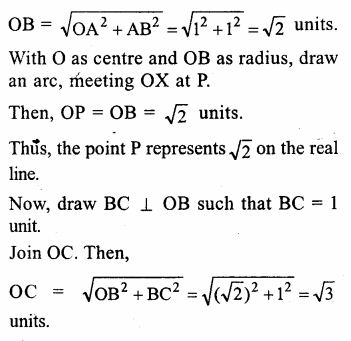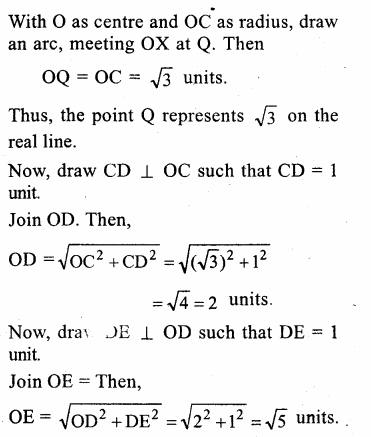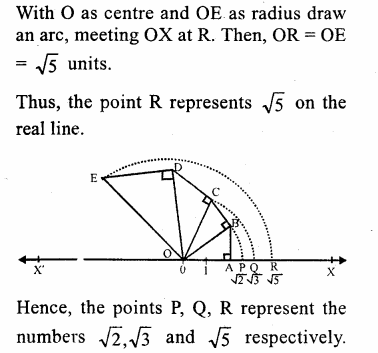Question 4.
Solution:
Firstly we represent √5 on the real line X’OX. Then we will find √6 and √7 on that real line.
Now, draw a horizontal line X’OX, taken as x-axis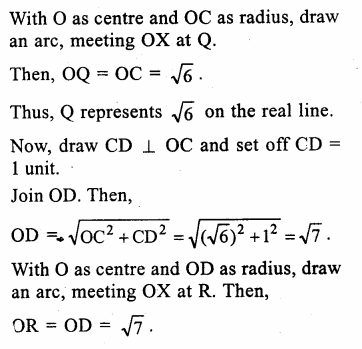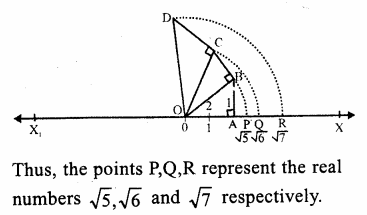Question 5.
Solution:
(i) 4 + √5 : It is irrational number because in it, 4 is a rational number and √5 is irrational and sum of a rational and an irrational is also an irrational.
(ii) (-3 + √6) It is irrational number because in it, -3 is a rational and √6 is irrational and sum or difference of a rational and irrational is an irrational.
(iii) 5√7 : It is irrational because 5 is rational and √7 is irrational and product of a rational and an irrational is an irrational.
(iv) -3√8 : It is irrational because -3 is a rational and √8 is an irrational and product of a rational and an irrational is also an irrational.
(v) $$\frac { 2 }{ \sqrt { 5 } }$$ It is irrational because 2 is a rational and √5 is an irrational and quotient of a rational and an irrational is also an irrational.
(vi) $$\frac { 4 }{ \sqrt { 3 } }$$ It is irrational because 4 is a rational and √3 is an irrational number and quotient of a rational and irrational is also an irrational.

Question 6.
Solution:
(i) True.
(ii) False, as the sum of two irrational number is irrational is not always true.
(iii) True.
(iv) False, as the product of two irrational numbers is irrational is not always true.
(v) True.
(vi) True.
(vii) False as a real number can be either rational or irrational.

Hope given RS Aggarwal Solutions Class 9 Chapter 1 Real Numbers Ex 1C are helpful to complete your math homework.

If you have any doubts, please comment below. Learn Insta try to provide online math tutoring for you.